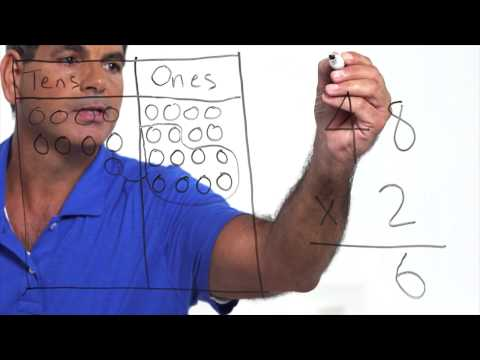# Patterns in powers of 10 (Full video)

Description: Sal examines patterns in powers of 10. He also introduces the terms exponent and base. Created by Sal Khan. So let's think about what happens when we multiply by 10, and see if we can see some type of a pattern. So let's just start with 1 and multiply that 1 by 10. Well we already know that 1 times 10 times 10 is 100, and then we're going to multiply that times 10 again.

### Other videos you might be interested in### Math Studio Talk: Instruction for 5.NBT (Full video)

#### EngageNY